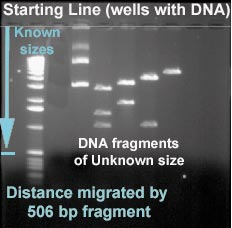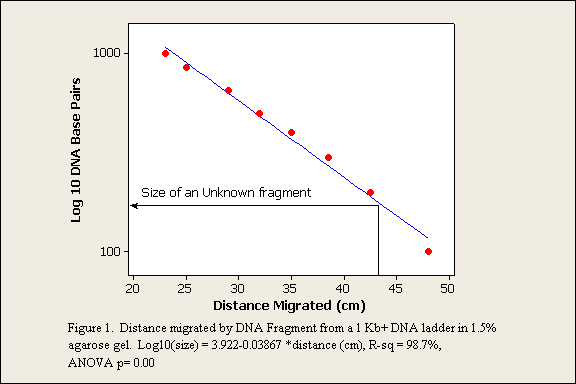Fragments of DNA were seperated by running them through a gel. The column on the left contains fragments of known sizes. The fragments have a distinct pattern that makes it possible to identify them. The distance the fragments of known size migrate is measured from the starting line.Then a standard curve is created to relate fragment size to distance migrated (Figure 1). The size of unknown fragments can be determined if the distance they migrated is measured. The equation for the regression line that defines the relationship can also be used to determine unknown fragment sizes(Figure 1). When the equation is used the result is the log 10 base pairs. To convert to base pairs the antilog must be calculated.